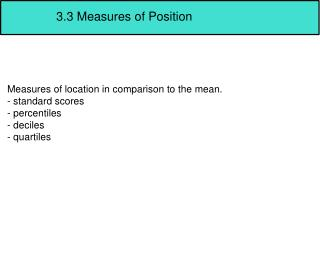# 3.3 Measures of Position - PowerPoint PPT PresentationDownload Presentation3.3 Measures of Position

3.3 Measures of PositionDownload Presentation## 3.3 Measures of Position

- - - - - - - - - - - - - - - - - - - - - - - - - - - E N D - - - - - - - - - - - - - - - - - - - - - - - - - - -
##### Presentation Transcript

1. 3.3 Measures of Position Measures of location in comparison to the mean. - standard scores - percentiles - deciles - quartiles

2. Z score or Standard Score 90 on a music test vs 45 on an English test The Z score tells us how many standard deviations a data value is above or below the mean.

3. Z score or Standard Score The Z score tells us how many standard deviations a data value is above or below the mean. -Subtract the mean from the value and divide by standard deviation Z = population sample Z=

4. A student scores a 65 on a calc test that has a mean of 50 and a standard deviation of 10. She scored a 30 on a history test with a mean of 25 and standard deviation of 5. Compare her relative positions on the two tests.

5. Test 1: X = 38, X = 40 and s = 5 Test 2: X = 94, X = 100, s = 10

6. A set of data has a mean of 105 and a standard deviation of 8. Find the data values that correspond to the following z scores. a. 2 b. -1 c. 0 d. -1.6

7. Percentiles Percentiles divide the data set into 100 equal groups

8. Percentiles are symbolized by P1,P2, P3, ...P100 Dividing the distribution into 100 groups. P99 P2 P1 1% 1% 1%

9. Percentiles The percentile corresponding to a given value X is found using the following formula: (number of values below X) + .5 100% Percentile = total number of values

10. A teacher gives a 20 point test to 10 students. Find the percentile rank of the score of 12. Then find the percentile rank of the score of 6. 18,15,12,6,8,2,3,5,20,10

11. 18,15,12,6,8,2,3,5,20,10 Now use the data to determine the value corresponding with the 25th percentile. n = # values p = percentile Formula to use: c = n*p 100 if c is not a whole number: round up to the nearest whole number if c is a whole number find the value halfway between the cth term and the c+1 term

12. Find the value that corresponds to the 60th percentile 2,3,5,6,8,10,12,15,18,20

13. The frequency distribution for the systolic blood pressure readings (in mm or mercury) of 200 randomly selected college students is shown here. Construct a Percentile Graph.

14. Cumulative Percentages Class Boundaries

15. Quartiles Quartiles divide the distribution into 4 groups: Q1,Q2,Q3, Q1 --> is the same as P25 or 25th percentile Q2 --> is the same as P50 or 50th percentile Q3 --> is the same as P75 or 75th percentile

16. MD largest data value smallest data value Q1 Q2 Q3 25% 25% 25% 25%

17. Finding the Quartiles Q2 --> The Median! Q1 --> The median of the data below Q2 Q3 --> The median of the data above Q2

18. Find Q1,Q2 and Q3 for the following data set. 15,13,6,5,12,50,22,18

19. Deciles Deciles divide the data into ______ groups. We can use the formula for Percentiles to find Deciles

20. Interquartile Range Quartiles can be used as a rough measurement of variability Interquartile Range: (IQR) the difference between Q3 and Q1. Or the range of the middle 50% of the data. We can use the IQR to identify outliers

21. Outlier An extremely high or extremely low value when compared to the rest of the data. Outliers affect: - mean -standard deviation -range

22. How do we determine if a value is high or low enough to be an outlier? 1. Put the data in order and find the quartiles. 2. Find IQR 3. Multiply the IQR by 1.5 4. Subtract the product from Q1 and add it to Q3. 5. If there are any values lower or higher than those two values, they are considered outliers.

23. Check the following for outliers 5,6,12,13,15,18,22

24. Using the Calculator 1. Enter data into L1 2. Press stat 3. Move the arrow 1 right to Calc 4. Press 1 for Var-Stats 5. Press 2nd L1 then enter

25. Using the Calculator Your calculator will display the following: x: sample mean x: sum of the data values x2: sum of the squares of the data values Sx: sample standard deviation : population standard deviation minX: smallest data value Q1: lower quartile Med: median Q3: upper quartile maxX: largest data value

26. Using the Calculator Use your calculator to find the stats on the following data: 11.2, 11.9, 12.0, 12.8, 13.4, 14.3

27. Using the Calculator For grouped data... 1. Enter midpoints into L1 2. Enter frequencies into L2 3. Press stat button 4. Use arrow to move 1 right to calc 5. Press 1 for vars stats 6. Press 2nd L1 and 2nd L2 then enter

28. Using the Calculator Find the mean and standard deviation of the following data.

29. Using the Calculator Graph a percentile graph on your calculator